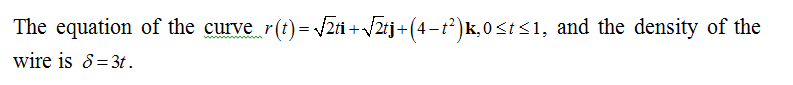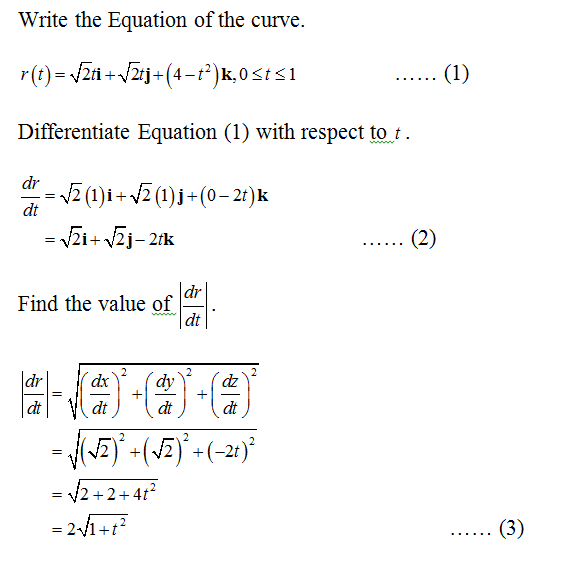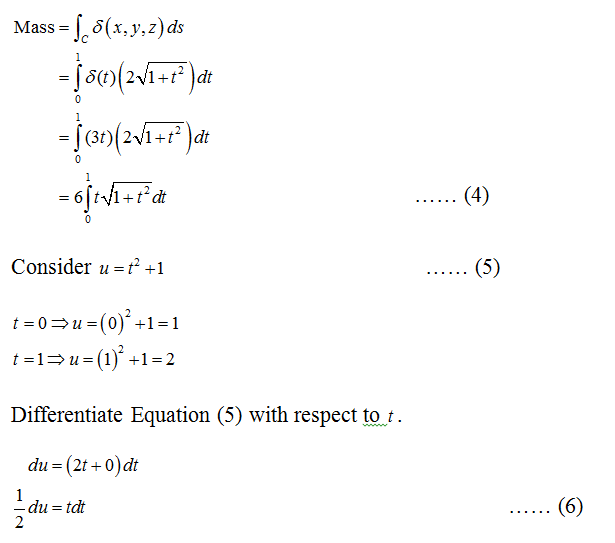# Find the mass of a thin wire lying along the curve r(t) = sqrt(2t) i + sqrt(2t) j + (4 - t2)k, 0<= t<= 1, if the density at t is (a) d = 3t and (b) d = 1.

Question
1 views

Find the mass of a thin wire lying along the curve r(t) = sqrt(2t) i + sqrt(2t) j + (4 - t2)k, 0<= t<= 1, if the density at t is (a) d = 3t and (b) d = 1.

check_circle

(a) Given information:Calculation:Find the Mass of the wire....

### Want to see the full answer?

See Solution

#### Want to see this answer and more?

Solutions are written by subject experts who are available 24/7. Questions are typically answered within 1 hour.*

See Solution
*Response times may vary by subject and question.
Tagged in